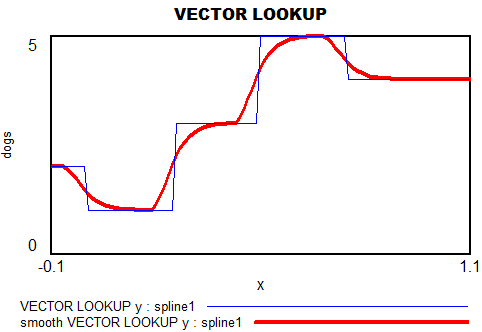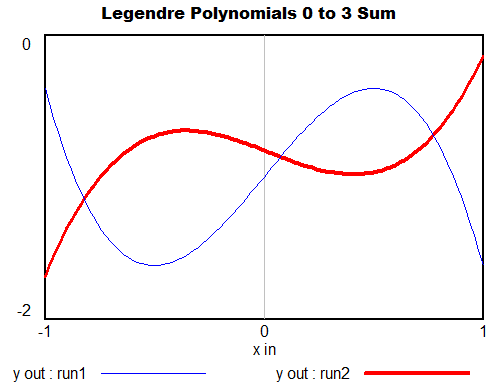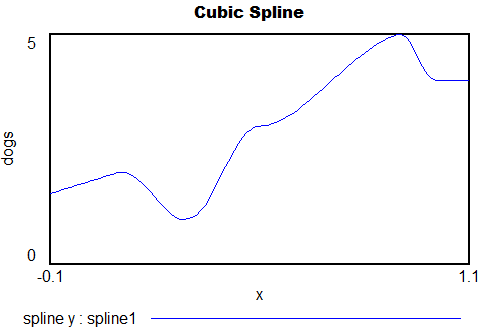## Polynomials & Interpolating Functions for Decision Rules

Sometimes it’s useful to have a way to express a variable as a flexible function of time, so that you can find the trajectory that maximizes some quantity like profit or fit to data. A caveat: this is not generally the best thing to do. A simple feedback rule will be more robust to rescaling and uncertainty and more informative than a function of time. However, there are times when it’s useful for testing or data approximation to have an open-loop decision rule. The attached models illustrate some options.

If you have access to arrays in Vensim, the simplest is to use the VECTOR LOOKUP function, which reads a subscripted table of values with interpolation. However, that has two limitations: a uniform time axis, and linear interpolation.If you want a smooth function, a natural option is to pick a polynomial, like

y = a + b*t + c*t^2 + d*t^3 …

However, it can be a little fiddly to interpret the coefficients or get them to produce a desired behavior. The Legendre polynomials provide a basis with nicer scaling, which still recovers the basic linear, quadratic, cubic (etc.) terms when needed. (In terms of my last post, their improved properties make them less sloppy.)You can generalize these to 2 dimensions by taking tensor products of the 1D series. Another option is to pick the first n terms of Pascal’s triangle. These yield essentially the same result, and either way, things get complex fast.

Back to 1D series, what if you want to express the values as a sequence of x-y points, with smooth interpolation, rather than arcane coefficients? One option is the Lagrange interpolating polynomial. It’s simple to implement, and has continuous derivatives, but it’s an N^2 problem and therefore potentially compute-intensive. It might also behave badly outside its interval, or inside due to ringing.

Probably the best choice for a smooth trajectory specified by x-y points (and optionally, the slope at each point) is a cubic spline or Bezier curve.Polynomials1.mdl – simple smooth functions, Legendre, Lagrange and spline, runs in any version of Vensim

InterpolatingArrays.mdl InterpolatingArrays.vpm – array functions, VECTOR LOOKUP, Lagrange and spline, requires Pro/DSS or the free Reader

## Data variables or lookups?

System dynamics models handle data in various ways. Traditionally, time series inputs were embedded in so-called lookups or table functions (DYNAMO users will remember TABHL for example). Lookups are really best suited for graphically describing a functional relationship. They’re really cool in Vensim’s Synthesim mode, where you can change the shape of a relationship and watch the behavioral consequence in real time.

Time series data can be thought of as f(time), so lookups are often used as data containers. This works decently when you have a limited amount of data, but isn’t really suitable for industrial strength modeling. Those familiar with advanced versions of Vensim may be aware of data variables – a special class of equation designed for working with time series data rather than endogenous structure.

There are many advantages to working with data variables:

• You can tell where there are data points, visually on graphs or in equations by testing for a special :NA: value indicating missing data.
• You can easily determine the endpoints of a series and vary the interpolation method.
• Data variables execute outside the main sequence of the model, so they don’t bog down optimization or Synthesim.
• It’s easier to use diverse sources for data (Excel, text files, ODBC, and other model runs) with data variables.
• You can see the data directly, without creating extra variables to manipulate it.
• In calibration optimization, data variables contribute to the payoff only when it makes sense (i.e., when there’s real data).

I think there are just two reasons to use lookups as containers for data:

• You want compatibility with Vensim PLE (e.g., for students)
• You want to expose the data stream to quick manipulation in a user interface

Otherwise, go for data variables. Occasionally, there are technical limitations that make it impossible to accomplish something with a data equation, but in those cases the solution is generally a separate data model rather than use of lookups. More on that soon.